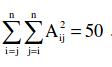##### Which of the above statements about eigenvalues of A is/are necessarily CORRECT?

Let A be n×n real valued square symmetric matrix of rank 2 with. Consider the following statements.

(I) One eigenvalue must be in [−5,5]
(II) The eigenvalue with the largest magnitude must be strictly greater than 5

Which of the above statements about eigenvalues of A is/are necessarily CORRECT?

(A) Both (I) and (II)

(B) (I) only

(C) (II) only

(D) Neither (I) nor (II)

Sumit Verma
28 Apr 2017 01:44 pm

Few words in the question are repeating. Plz correct.

shweta
28 Apr 2017 03:55 pm

corrected .. now plz solve..

Sumit Verma
28 Apr 2017 07:00 pm

Note two things in the question:
1. Matrix is symmetric.
2. Rank of matrix is 2.
Matrix is of order nxn so n-2 eigen  values will be zero.
So eigen values will be, e1, e2, 0, 0, 0......
We have,. = Trace of (A . AT)  = Trace of (A2)  = e12 + e22 +0+0......
Hence e12 + e22 = 50.
So with the above equation, it can be determined that one eigenvalue must be in [−5,5].
Hence B is the correct option.

shweta
28 Apr 2017 09:16 pm

Matrix is of order nxn so n-2 eigen  values will be zero.
So eigen values will be, e1, e2, 0, 0, 0......

how??????

Sumit Verma
28 Apr 2017 11:56 pm

@shweta1920 Matrix is of order nxn so we will get auxiliary equation of order n and roots of this equation will be eigen value. Here it is given that there are only two eigen values, so you can say that all the other roots(eigen values) will be zero.